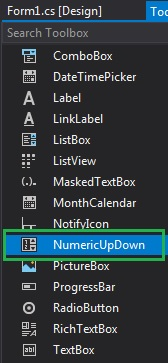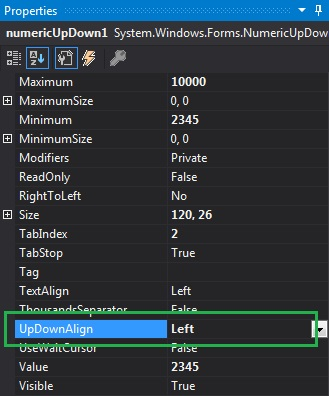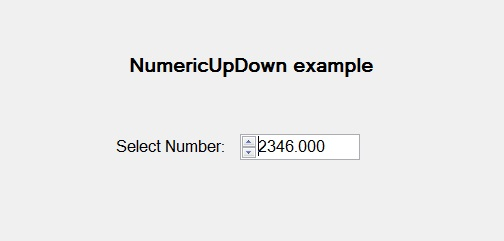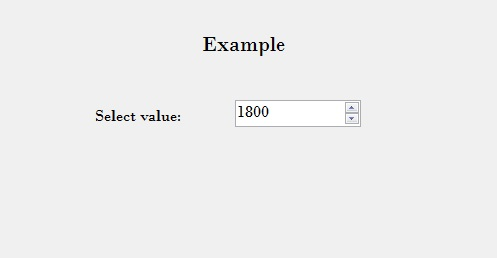# How to set Alignment of Up and Down buttons of NumericUpDown in C#?

• Last Updated : 29 Jul, 2019

In Windows Forms, NumericUpDown control is used to provide a Windows spin box or an up-down control which displays the numeric values. Or in other words, NumericUpDown control provides an interface which moves using up and down arrow and holds some pre-defined numeric value. In NumericUpDown control, you can set the alignment of the up and down buttons of the up-down control using UpDownAlign Property. This property has two values and these values are defined under LeftRightAlignment enum and the values are:

• Left: It aligns the up and down button on the left-hand side of the NumericUpDown control.
• Right: It aligns the up and down button on the right-hand side of the NumericUpDown control.

The default value of this property is right. You can set this property in two different ways:

1. Design-Time: It is the easiest way to set the alignment of the up and down buttons of the NumericUpDown as shown in the following steps:

• Step 1: Create a windows form as shown in the below image:

Visual Studio -> File -> New -> Project -> WindowsFormApp• Step 2: Next, drag and drop the NumericUpDown control from the toolbox on the form as shown in the below image:• Step 3: After drag and drop you will go to the properties of the NumericUpDown and set the alignment of the up and down buttons of the NumericUpDown as shown in the below image:Output:2. Run-Time: It is a little bit trickier than the above method. In this method, you can set the alignment of the up and down buttons of the NumericUpDown control programmatically with the help of given syntax:

`public System.Windows.Forms.LeftRightAlignment UpDownAlign { get; set; }`

Here, LeftRightAlignment represents the value of this property. It will throw an InvalidEnumArgumentException if the values do not belong to the LeftRightAlignment enum. The following steps show how to set the alignment of the up and down buttons of the NumericUpDown dynamically:

• Step 1: Create a NumericUpDown using the NumericUpDown() constructor is provided by the NumericUpDown class.
```// Creating a NumericUpDown
NumericUpDown n = new NumericUpDown();
```
• Step 2: After creating NumericUpDown, set the UpDownAlign property of the NumericUpDown provided by the NumericUpDown class.
```// Setting the UpDownAlign property
n.UpDownAlign = LeftRightAlignment.Right;
```
• Step 3: And last add this NumericUpDown control to the form using the following statement:
```// Adding NumericUpDown control on the form
```

Example:

 `using` `System;``using` `System.Collections.Generic;``using` `System.ComponentModel;``using` `System.Data;``using` `System.Drawing;``using` `System.Linq;``using` `System.Text;``using` `System.Threading.Tasks;``using` `System.Windows.Forms;`` ` `namespace` `WindowsFormsApp44 {`` ` `public` `partial` `class` `Form1 : Form {`` ` `    ``public` `Form1()``    ``{``        ``InitializeComponent();``    ``}`` ` `    ``private` `void` `Form1_Load(``object` `sender, EventArgs e)``    ``{``        ``// Creating and setting the``        ``// properties of the labels``        ``Label l1 = ``new` `Label();``        ``l1.Location = ``new` `Point(348, 61);``        ``l1.Size = ``new` `Size(215, 25);``        ``l1.Text = ``"Example"``;``        ``l1.Font = ``new` `Font(``"Bodoni MT"``, 16);``        ``this``.Controls.Add(l1);`` ` `        ``Label l2 = ``new` `Label();``        ``l2.Location = ``new` `Point(242, 136);``        ``l2.Size = ``new` `Size(103, 20);``        ``l2.Text = ``"Select value:"``;``        ``l2.Font = ``new` `Font(``"Bodoni MT"``, 12);``        ``this``.Controls.Add(l2);`` ` `        ``// Creating and setting the``        ``// properties of NumericUpDown``        ``NumericUpDown n = ``new` `NumericUpDown();``        ``n.Location = ``new` `Point(386, 130);``        ``n.Size = ``new` `Size(126, 26);``        ``n.Font = ``new` `Font(``"Bodoni MT"``, 12);``        ``n.Minimum = 1800;``        ``n.Maximum = 3000;``        ``n.Increment = 1;``        ``n.UpDownAlign = LeftRightAlignment.Right;`` ` `        ``// Adding this control``        ``// to the form``        ``this``.Controls.Add(n);``    ``}``}``}`

Output:My Personal Notes arrow_drop_up